Next: Output of Fourier Transforms Up: Storage: What Goes Where Previous: Fourier Transform Definitions and

### Output of Fourier Transforms of Complex Data

The output of fftc1 is arranged as follows. The first half of the output array represents the positive frequencies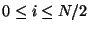. The index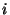then wraps around toand begins ascending from there. (Remember thatand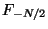are the same point.) Bearing in mind that the array contains alternating real and imaginary components, the input and output arrays look like: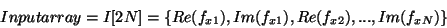(7)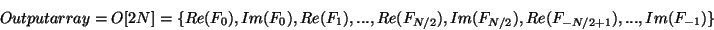(8)

Remember thatand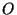are actually the same array because the output data are written over the original input array. The output of fftcn is arranged analogously to fftc1. Each dimension goes from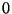to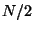and then fromup to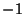and the whole array alternates between real and imaginary parts.

To do an inverse Fourier transform simply arrange the valuesas described here. If the arraywas produced as output of the FFTEASY routines then it is already arranged correctly to be put back in for the inverse Fourier transform.Next: Output of Fourier Transforms Up: Storage: What Goes Where Previous: Fourier Transform Definitions and